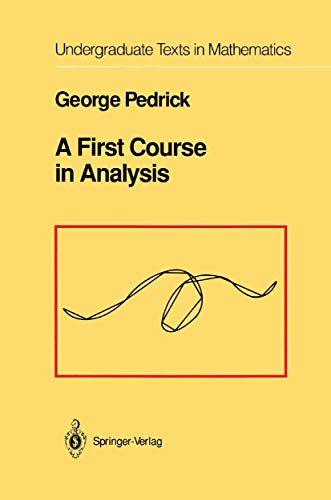• Free Shipping on all orders in Australia
• Over 7 million books in stock
• Proud to be B-Corp
• We aim to be carbon neutral by 2022
• Over 120,000 Trustpilot reviews
Item 1 of 0# A First Course in Analysis by George Pedrick

Condition - New
\$110.29
Only 2 left

## Summary

This text on advanced calculus discusses such topics as number systems, the extreme value problem, continuous functions, differentiation, integration and infinite series. The book is intended both for a terminal course and as preparation for more advanced studies in mathematics, science, engineering and computation.

## A First Course in Analysis Summary

### A First Course in Analysis by George Pedrick

This text on advanced calculus discusses such topics as number systems, the extreme value problem, continuous functions, differentiation, integration and infinite series. The reader will find the focus of attention shifted from the learning and applying of computational techniques to careful reasoning from hypothesis to conclusion. The book is intended both for a terminal course and as preparation for more advanced studies in mathematics, science, engineering and computation.

Background Number Systems.- 1. Counting: The Natural Numbers.- 2. Measurement: The Rational Numbers.- The Axioms of Ordered Fields.- 3. Decimal Representation. Irrationals.- I ANALYSIS.- 1 Approximation: The Real Numbers.- 1. Least Upper Bound.- 2. Completeness. Nested Intervals.- 3. Bounded Monotonic Sequences.- 4. Cauchy Sequences.- 5. The Real Number System.- 6. Countability.- Appendix. The Fundamental Theorem of Algebra. Complex Numbers.- 2 The Extreme-Value Problem.- 1. Continuity, Compactness, and the Extreme-Value Theorem.- 2. Continuity of Rational Functions. Limits of Sequences.- Appendix. Completion of the Proof of the Fundamental Theorem of Algebra.- 3. Sequences and Series of Reals. The Number e.- 4. Sets of Reals. Limits of Functions.- 3 Continuous Functions.- 1. Implicit Functions,$$\sqrt[n]{x}$$The Intermediate-Value Theorem.- 2. Inverse Functions. xr for r ? ?.- 3. Continuous Extension. Uniform Continuity. The Exponential and Logarithm.- 4. The Elementary Functions.- 5. Uniformity. The Heine-Borel Theorem.- 6. Uniform Convergence. A Nowhere Differentiable Continuous function.- 7. The Weierstrass Approximation Theorem.- Summary of the Main Properties of Continuous Functions.- Appendix. A Space-Filling Continuous Curve.- II FOUNDATIONS OF CALCULUS.- 4 Differentiation.- 1. Differential and Derivative. Tangent Line.- 2. The Foundations of Differentiation.- 3. Curve Sketching. The Mean-Value Theorem.- 4. Taylor's Theorem.- 5. Functions Defined Implicitly.- 5 Integration.- 1. Definitions. Darboux Theorem.- 2. Foundations of Integral Calculus. The Fundamental Theorem of Calculus.- 3. The Nature of Integrability. Lebesgue's Theorem.- 4. Improper Integral.- 5. Arclength. Bounded Variation.- A Word About the Stieltjes Integral and Measure Theory.- 6 Infinite Series.- 1. The Vibrating String.- 2. Convergence: General Considerations.- 3. Convergence: Series of Positive Terms.- 4. Computation with Series.- 5. Power Series.- 6. Fourier Series.

NLS9781461264354
9781461264354
1461264359
A First Course in Analysis by George Pedrick
New
Paperback
Springer-Verlag New York Inc.
2012-09-05
279
N/A
Book picture is for illustrative purposes only, actual binding, cover or edition may vary.
This is a new book - be the first to read this copy. With untouched pages and a perfect binding, your brand new copy is ready to be opened for the first time